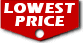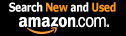# Introductory Discrete Mathematics (Dover Books on Computer Science) / V. K . Balakrishnan,

List Price: \$14.95
Our Price: \$14.95For Bulk orders
Quantity

Used Book Price:
/ V. K . Balakrishnan,
Publisher: Dover Publications
Availability:
Sales Rank: 346219
 ISBN-10: 0486691152ISBN-13: 9780486691152

This concise text offers an introduction to discrete mathematics for undergraduate students in computer science and mathematics. Mathematics educators consider it vital that their students be exposed to a course in discrete methods that introduces them to combinatorial mathematics and to algebraic and logical structures focusing on the interplay between computer science and mathematics. The present volume emphasizes combinatorics, graph theory with applications to some stand network optimization problems, and algorithms to solve these problems.
Chapters 0 3 cover fundamental operations involving sets and the principle of mathematical induction, and standard combinatorial topics: basic counting principles, permutations, combinations, the inclusion-exclusion principle, generating functions, recurrence relations, and an introduction to the analysis of algorithms. Applications are emphasized wherever possible and more than 200 exercises at the ends of these chapters help students test their grasp of the material.
Chapters 4 and 5 survey graphs and digraphs, including their connectedness properties, applications of graph coloring, and more, with stress on applications to coding and other related problems. Two important problems in network optimization the minimal spanning tree problem and the shortest distance problem are covered in the last two chapters. A very brief nontechnical exposition of the theory of computational complexity and NP-completeness is outlined in the appendix.

Now you can buy Books online in USA,UK, India and more than 100 countries.
*Terms and Conditions apply
Disclaimer: All product data on this page belongs to.
No guarantees are made as to accuracy of prices and information.